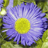# Calculate mileage calculation in model builder:

630
6
10-19-2011 11:23 AMNew Contributor II
Hello:
I am attempting to calculate linear mileage of stream data for three predefined fields in model builder.  The conceptual approach is to calculate all segments mileage in the first field (total_miles), then calculate a fraction of the records in another field (need_miles), and finally, calculate the percentage of the two in the final field (percentage).  I'll use frequency to agregate the segments to their respective HUC 12 areas.  The problem I am having, is how to calculate geometry (linear mileage) in model builder?  I can't seem to locate the tool that may be dragged to model builder.  Thanks so much in advance!!!!
Tags (3)
6 RepliesbyMVP Honored Contributor
Hello:
I am attempting to calculate linear mileage of stream data for three predefined fields in model builder.  The conceptual approach is to calculate all segments mileage in the first field (total_miles), then calculate a fraction of the records in another field (need_miles), and finally, calculate the percentage of the two in the final field (percentage).  I'll use frequency to agregate the segments to their respective HUC 12 areas.  The problem I am having, is how to calculate geometry (linear mileage) in model builder?  I can't seem to locate the tool that may be dragged to model builder.  Thanks so much in advance!!!!

A few ways to do this.  Sum the Length value in the frequency in whatever units they are listed (assming this is a geodatabase and not a shapefile), then do the conversion to miles at the join and calculation stage.  If you are using a shapefile, first calculate a field called MILES using the Field Calculator tool.  The python calculation to get the length of a line and make the appropriate units conversion is:

Parser:  Python

Pre-Logic Code:
def Output(Length):
return Length / 5280 # assumes that shape length is in Feet and is being converted to Miles.

Expression:
Output(!SHAPE.LENGTH!)

now sum the MILES field values in your frequency and you can get the all of the fields filled in that you need.New Contributor II
Thanks for you quick reply, although am not certain I'm following your logic precisely.  And yes, I am using feature classes.  When calculating without model builder, I create the two fields to house mileage (total miles and need miles), use calculate geometry to populate the segmets mileage, and then perform frequency analysis to summarize the values of the HUC 12s.  Using model builder,  should I be using calculate field prior to utilizing frequency, and what calculation would work for empty fields? Thanks again!byMVP Honored Contributor
Thanks for you quick reply, although am not certain I'm following your logic precisely.  And yes, I am using feature classes.  When calculating without model builder, I create the two fields to house mileage (total miles and need miles), use calculate geometry to populate the segmets mileage, and then perform frequency analysis to summarize the values of the HUC 12s.  Using model builder,  should I be using calculate field prior to utilizing frequency, and what calculation would work for empty fields? Thanks again!

I overcomplicated the calculation.  It can be done as a simple calculation:

Parser:  Python

Expression: !SHAPE.LENGTH! / 5280 # assumes base units is in feet and you want miles.

The above calculation is done using the Field Calculator tool and does what the Calculate Geometry tool does in ArcMap, so you would use it the same way that you use the Calculate Geometry tool in your desktop process.  This Field Calculator expression is what you had to do in ArcMap versions that existed when you had no Calculate Geometry option.  With the Total_Miles and Need_Miles values calculated you would run the Frequency tool the same way you do now.

You should run a calculation on Need_Miles to populate it with 0 on all records that are Null and that you do not want to be in the subset that has Mileage calcuated into the Need_Miles field prior to running Frequency, unless you find that the Frequency tool does what you want when these values are Null.New Contributor II
Thanks Richard!  You rock!  I'll give her a try...New Contributor II
Richard, the field calculation for !SHAPE.LENGTH! / 5280 # does not work.  It produces a syntax error when ran on an empty field through field calculation.  Any thoughts?byMVP Honored Contributor
Richard, the field calculation for !SHAPE.LENGTH! / 5280 # does not work.  It produces a syntax error when ran on an empty field through field calculation.  Any thoughts?

What version of ArcGIS are you using and are you doing this in Desktop or with Model Builder.  This should work for ArcGIS 9.3 and up, although you may have to change the case of the words to mixed case for 10 when ESRI started to make Python case sensitive.  At 10 you can do:

!Shape.Length@miles! # to get miles

At 9.2 I think you can only use Python in Model Builder and the syntax might be !Shape!.Length or some other variant.  Check the help files, since there should be something on this for 9.2.  If you will not be exporting to a Python script and running it directly in Model Builder you can use VBA in an advanced calculation at 9.2, where the syntax would be:

Parser: VBA

Pre-Logic Code:
Dim dblLength as double
Dim pCurve as ICurve
Set pCurve = [SHAPE]
dblLength = pCurve.Length / 5280

Expression: dblLength